#### Thank you for registering.

One of our academic counsellors will contact you within 1 working day.

Click to Chat

1800-5470-145

+91 7353221155

CART 0

• 0
MY CART (5)

Use Coupon: CART20 and get 20% off on all online Study Material

ITEM
DETAILS
MRP
DISCOUNT
FINAL PRICE
Total Price: Rs.

There are no items in this cart.
Continue Shopping

# how do i this kind of ques ?i think that conservation of energy is required in this ques. but I am not able to apply it.please help me.Shobhit Varshney IIT Roorkee
6 years ago
Hi,

When the acceleration at P is given, the spring would start to stretch till the the force in the spring becomes m*4. So, the maximum acceleration of the block will be 4 m/s2
There is no use of conservation of energy in this question.

thanks
6 years ago
p { margin-bottom: 0.25cm; line-height: 120%; }

Let relaxed length of the spring is L. Let coordinates of block is x1, coordinates of point P is x2 and elongation of the spring is x at certain time t. Then we can write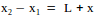Differentiating twice wrt t, we can write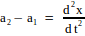(1)

Given that acceleration of P is a2 (= 4 m/s2), and acceleration of the block is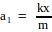We can write the above equation as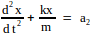(2)

Solution of this differential equation gives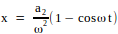, where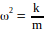Acceleration a1 of the block is given by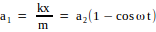(3)

To find maximum and minimum values of a1,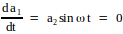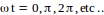From this we can easily see that a1 will have minimum value when ωt = 0, 2π, etc and will be maximum at ωt = π, 3π, etc..

Hence from equation 3, minimum value of a1 = 0, whereas maximum value of a1 = 8 m/s2

6 years ago
p { margin-bottom: 0.25cm; line-height: 120%; }

Alternatively, let us try to solve this problem from the reference frame of point P. Viewed from this frame, there will be a pseudo force -map acting on the mass m, where ap is the accleration of P. You can see that since in this frame point P is fixed, the mass m will have Simple Harmonic Oscillations as it is attached to the spring.

I am assuming that you are aware of Simple Harmonic Motion (SHM) concepts, then you will know that maximum acceleration of the mass will happen at extreme position (maximum extension).

At the maximum extension position, the mass will come to instantaneous rest, and work done by the pseudo force will be equal to the potential energy stored in the spring.

So, if x is the maximum extension, then we can write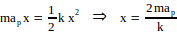and at the maximum extension, acceleration of the mass is given by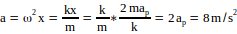, as ap = 4 m/s2, given.

Hope this helps.